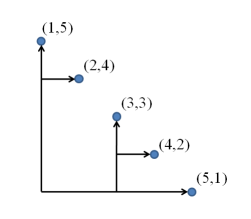시간 제한 메모리 제한 제출 정답 맞은 사람 정답 비율
2 초 512 MB 0 0 0 0.000%

## 문제

Consider a two-dimensional space with a set of points (xiyi) that satisfy xi < xj and yi > yj for all i < j. We want to have them all connected by a directed tree whose edges go toward either right (x positive) or upward (y positive). The figure below shows an example tree.Figure 1: An example tree

Write a program that finds a tree connecting all given points with the shortest total length of edges.

## 입력

The input begins with a line that contains an integer n (1 ≤ n ≤ 1000), the number of points. Then n lines follow. The i-th line contains two integers xi and yi (0 ≤ xiyi ≤ 10000), which give the coordinates of the i-th point.

## 출력

Print the total length of edges in a line.

## 예제 입력 1

5
1 5
2 4
3 3
4 2
5 1


## 예제 출력 1

12


## 예제 입력 2

1
10000 0


## 예제 출력 2

0